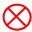In other languages:

# User:Takenm/Lab/ja

(diff) ← Older revision | Latest revision (diff) | Newer revision → (diff)Takenm/Lab Edit
 マップ上での表示色 耐久力 150 最大スタック数 10 寸法 3×3 エネルギー消費量 60 kW (電気) 掘削時間 0.2 モジュールスロット 2 slots Prototype type Internal name lab 必要なテクノロジー 不要 研究可能 製作可能設備Science packs put into the front lab will be passed to labs behind it.

When productivity modules are used in labs, the productivity bonus is directly calculated and applied each tick so the productivity bar is simply cosmetic. This means that it does not matter that the productivity bar resets when the research is changed, no productivity bonus is lost. 

プレイヤーは一度に一つのテクノロジーしか研究できませんが、研究所を使うことでより早くテクノロジーを研究終えることができます。研究速度ボーナスとモジュールによるボーナスは次の式で計算することができます：`研究速度ボーナス × モジュールによるボーナス = 速度ボーナス`。この式ではボーナスのパーセンテージは整数の比に置き換えて（例えば +140%であれば2.4）計算します。

## Production requirements

Calculating the number of science packs needed per second is straightforward:

• `ERS = (1 + B[r] ÷ 100) × (1 + M[r] ÷ 100)`
• `ACT = T[r] ÷ ERS`
• `PPS = N ÷ ACT`

Where:

• ERS is "effective lab research speed"
• B[r] is the Lab Research Speed bonus as reported by the game, in percent
• M[r] is the sum of all module speed effects (Speed modules - positive; Productivity modules - negative), in percent
• ACT is "adjusted cycle time"
• T[r] is the research cycle time as displayed in the research screen
• PPS is "packs per second"
• N is the number of labs available.

Thus, for a 10-lab setup, researching Nuclear Power (30 second cycle time) with Lab Research Speed 4 (140% bonus) and no module effects, the calculation is:

• `ERS = 1 + (140 ÷ 100) = 2.4`
• `ACT = 30 ÷ 2.4 = 12.5 s`
• `PPS = 10 ÷ 12.5 = 0.8`

This means 0.8 science packs per second, of each type, would need to be produced to continuously supply the labs.

### Equation simplification

Assuming all labs have the same B[r] (which they always will unless they are affected by different module configurations), the above calculations can be combined into one equation:

• `PPS = N × (1 + B[r] ÷ 100) × (1 + M[r] ÷ 100) ÷ T[r]`

Thus for the numerical example:

• `PPS = 10 × (1 + 140 ÷ 100) × 1 ÷ 30` = `(10 ÷ 30) × (1 + 1.4)` = ` (1 ÷ 3) × 2.4` = ` 2.4 ÷ 3` = `0.8` packs per second

If trying to find the amount of labs needed to consume a given amount of packs per second, use the following rearrangement:

• `N = ((1 + B[r] ÷ 100) × (1 + M[r] ÷ 100) ÷ T[r] ÷ PPS) ^ −1`

## History

• 0.15.12:
• Lab speed info in the description contains the researched speed bonus as well.
• 0.12.6:
• The research speed of a lab is now not dependent on its electricity consumption, and can be scripted.
• 0.12.0:
• Lab research is now continuous; Science packs now have progress bars.
• 0.9.2:
• Labs are now named after early access backers when built from blueprints.
• 0.7.2:
• Changed the recipe of Lab to require 4 transport belts, down from 5.
• 0.7.0:
• Added support for modules to labs.
• 0.6.0:
• New graphics.
• Labs are dedicated to backers (displayed in entity info).
• 0.2.7:
• Contents of the Lab is now shown in the entity info.